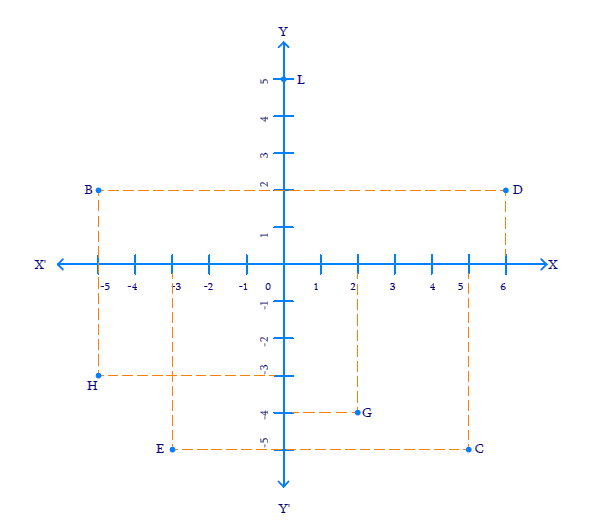# Ex.3.2 Q2 Coordinate Geometry Solution - NCERT Maths Class 9

Go back to  'Ex.3.2'

## Question

See the given figure, and write the following:

(i)The coordinates of $$B.$$

(ii) The coordinates of $$C.$$

(iii) The point identified by the coordinates ($$–3, –5$$).

(iv) The point identified by the coordinates ($$2, –4$$).

(v) The abscissa of the point $$D.$$

(vi) The ordinate of the point $$H.$$

(vii) The coordinates of the point $$L.$$

(viii)The coordinates of the point $$M.$$Video Solution
Coordinate Geometry
Ex 3.2 | Question 2

## Text Solution

Steps:

From the Figure above,

(i) The co-ordinates of point $$B$$ is the distance of point $$B$$ from $$x-$$axis and $$y-$$axis. Therefore, the co-ordinates of point $$B$$ are ($$–5, 2$$).

(ii) The co-ordinates of point $$C$$ is the distance of point $$C$$ from $$x-$$axis and $$y-$$axis. Therefore, the co-ordinates of point $$C$$ are ($$5, –5$$).

(iii) The point that represents the co-ordinates ($$–3, –5$$) is $$E.$$

(iv) The point that represents the co-ordinates ($$2, –4$$) is $$G.$$

(v) The abscissa of point $$D$$ is the distance of point $$D$$ from the $$y-$$axis. Therefore, the abscissa of point $$D$$ is $$6.$$

(vi)  The ordinate of point $$H$$ is the distance of point $$H$$ from the $$x-$$axis. Therefore, the abscissa of point $$H$$ is $$–3.$$

(vii)  The co-ordinates of point $$L$$ in the above figure is the distance of point $$L$$ from $$x-$$axis and $$y-$$axis. Therefore, the co-ordinates of point $$L$$ are ($$0, 5$$).

(viii)  The co-ordinates of point $$M$$ in the above figure is the distance of point $$M$$ from $$x-$$axis and $$y-$$axis. Therefore, the co-ordinates of point $$M$$ are ($$–3, 0$$).

Video Solution
Coordinate Geometry
Ex 3.2 | Question 2

Learn from the best math teachers and top your exams

• Live one on one classroom and doubt clearing
• Practice worksheets in and after class for conceptual clarity
• Personalized curriculum to keep up with school